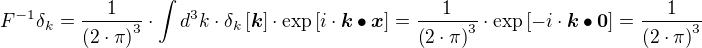Periodic functions can be expressed in their frequency domain.

First, the function (let's call it f) must be square integrable (at least over the periodic interval):Then a base can be found and the function be expressed using a (continuous) linear combination:This operation is called the inverse transform.The coefficientscan be calculated by:## Delta Distribution and Fourier Transform

The Fourier Transform can also be used with the δ Distribution:

First, it holds that:Proof.

This also works in the other direction (!!)Proof.

In Quantum Mechanics it is customary to instead use the momentum, in that case it's slightly different:Note that δ is not linear.

## Triple Triple Integrals

Sometimes multiple integrations can be simplified by observing that:Likewise:And for Quantum Mechanics: